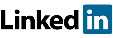Visibility into the FPGA.

# How many nodes can I sample continuously without creating overflows?

The number of nodes (or bits) that can be captured continuously with one single capture unit is function of the following parameters:
– The number of transceivers used to extract data : LinkNr – 1 to 4.
– The line rate (or ‘data rate’) : LinkDR – this parameter depends on the transceiver reference clock frequency and is chosen when setting up EXOSTIV IP – up to 12.5 Gbps.
– The frequency of the clock used for sampling the nodes from the FPGA: fSampling
– The fact that we use 98% of the bandwidth of each link for data.
– The grouping of data per 16 bits per link in EXOSTIV IP.

As a result, the number of bits (NrOfBits) that can be continuously sampled with a capture unit without creating overflows can be computed as follows:

## NrOfBits = LinkNr * INT[(LinkDR*0.98) / fSampling / 16] * 16

Example: